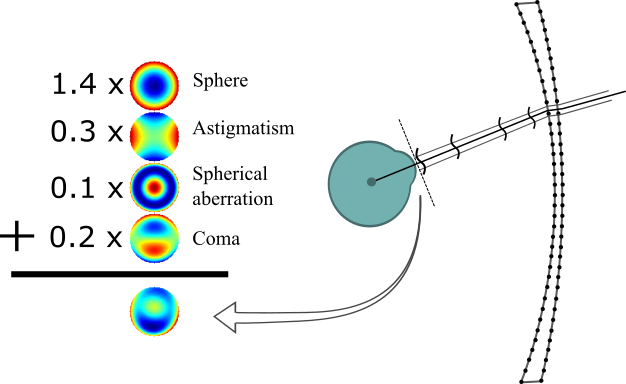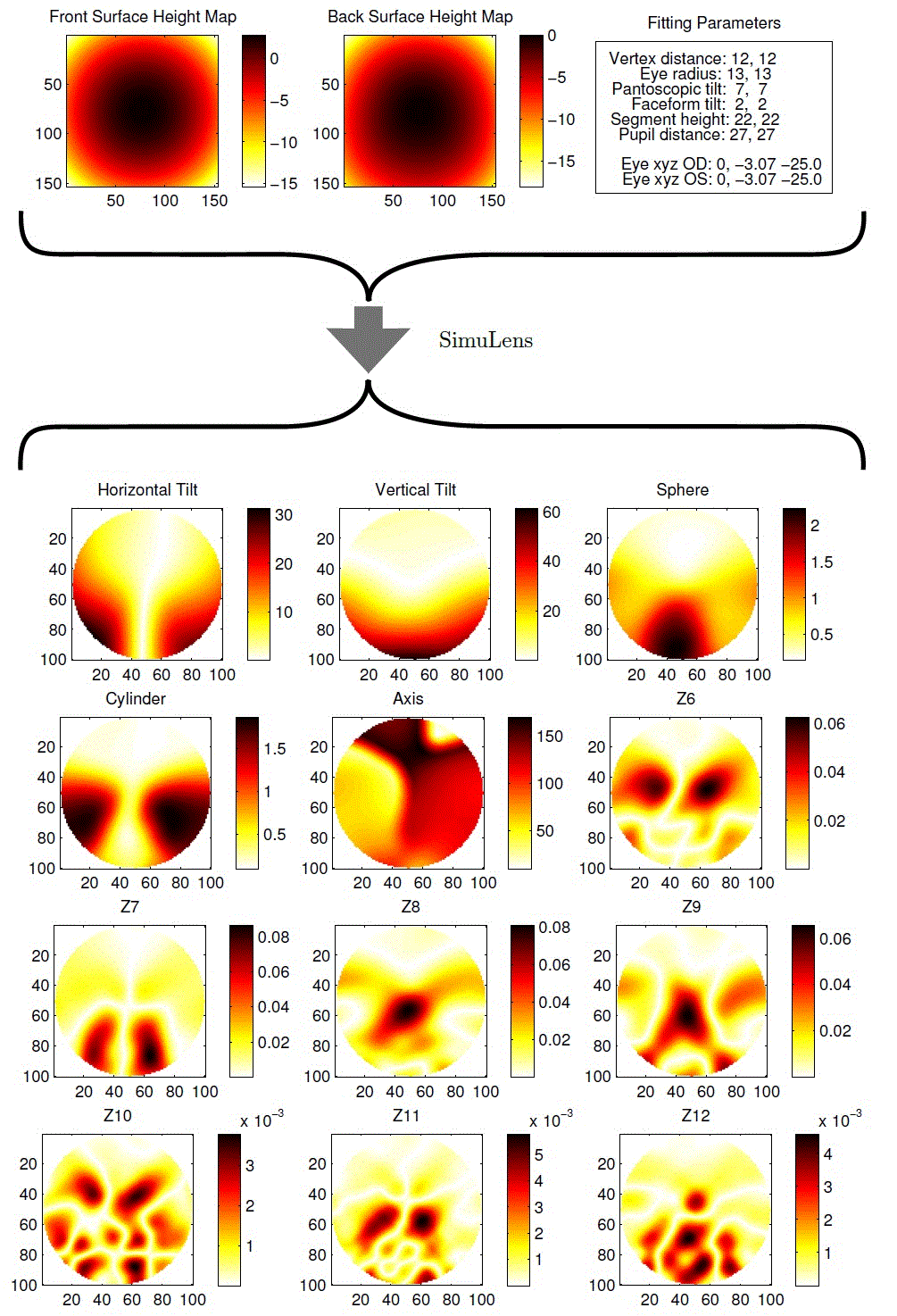# SimuLens

SimuLens performs a realistic simulation of a spectacle lens in use. The lens is to be described by arbitrary sets of points describing the front and back surfaces (e.g. generator points-files), and a refractive index. The eye position relative to the points is computed based on fitting parameters.

The more complete and accurate the information describing position is, the more accurate the estimate of visual performance will be. Ideally a complete set of of fitting parameters should be collected.

From the above information we compute XYZ locations of the eyes and surface points. Then SimuLens estimates lens performance for each gaze direction through the lens. A dense bundle of rays is propagated through each point on the lens, and used to estimate the total wavefront error at the cornea. This wavefront error measurement can be described as an expansion on Zernike polynomials.So SimuLens gives us a vector of coefficients for each point on the lens. This can also be viewed as a collection of images, each showing how a single coefficient–representing a component of the distortion–varies over the lens.